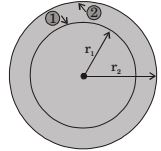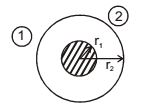## Heat Transfer Miscellaneous

#### Heat-Transfer

1. A solid sphere of radius r1 = 20 mm is placed concentrically inside a hollow sphere of radius r2 = 30 mm as shown in the figure.The view factor F21 for radiation heat transfer is

1. F11 + F12 = 1, here F11 = 0
∴ F12 = 1Now from reciprocating law
A1 F12 = A2 F21
∴ 22π (20)2 × 1 = 2π (30)2 × F21

 ⇒ F21 =102 = 4 30 9

##### Correct Option: B

F11 + F12 = 1, here F11 = 0
∴ F12 = 1Now from reciprocating law
A1 F12 = A2 F21
∴ 22π (20)2 × 1 = 2π (30)2 × F21

 ⇒ F21 =102 = 4 30 9

1. The heat loss from a fin is 6 W. The effectiveness and efficiency of the fin are 3 and 0.75 respectively. The heat loss (in W) from the fin, keeping the entire fin surface at base temperature is _____.

1.  ηfin = qactual qmaximum possible

 qmax = 6 = 8 W 0.75

##### Correct Option: B

 ηfin = qactual qmaximum possible

 qmax = 6 = 8 W 0.75

1. Which one of the following configurations has the highest fin effectiveness?

1. NA

##### Correct Option: A

NA

1. A fin has 5 mm diameter and 100 mm length. The thermal conductivity of fin material is 400 Wm–1K–1. One end of the fin is maintained at 130°C and its remaining surface is exposed to ambient air at 30°C. If the convective heat transfer coefficient is 40 Wm–2K–1, the heat loss (in W) from the fin is

1. Fin diameter, d = 5 mm
Fin length, L = 100 mm
k = 400 W/mK
T0 = 130°C
T = 30°C
h = 40 W/m2K
∴ Heat loss from fin Q = √kAhP (T0 - T) tanh ml
where P = πd = 3.14 × 5 × 10– 3 = 0.015

 A = π d2 = π × (5 × 10– 3)2 = 1.96 × 10– 5 m 2 4 4

∴ m = √hp / kA = √(40 × 0.0157) / (400 × 1.96 × 10– 5) = 8.949
∴ ml = 8.949 × 0.100 = 0.895
∴ Q = √40 × 0.0157 × 400 × 1.96 × 10– 5(T0 - T) tanh (0.895) = 5.0 W

##### Correct Option: B

Fin diameter, d = 5 mm
Fin length, L = 100 mm
k = 400 W/mK
T0 = 130°C
T = 30°C
h = 40 W/m2K
∴ Heat loss from fin Q = √kAhP (T0 - T) tanh ml
where P = πd = 3.14 × 5 × 10– 3 = 0.015

 A = π d2 = π × (5 × 10– 3)2 = 1.96 × 10– 5 m 2 4 4

∴ m = √hp / kA = √(40 × 0.0157) / (400 × 1.96 × 10– 5) = 8.949
∴ ml = 8.949 × 0.100 = 0.895
∴ Q = √40 × 0.0157 × 400 × 1.96 × 10– 5(T0 - T) tanh (0.895) = 5.0 W

1. Two rods, one of length L and the other of length 2L are made of the same material and have the same diameter. The two ends of the longer rod are maintained at 100°C. One end of the shorter rod is maintained at 100°C while the other end is insulated. Both the rods are exposed to the same environment at 40°C. The temp at the insulated end of the shorter rod is measured to be 55°C. The temperature at the mid point of the longer rod would be.

1. NA

NA